# Generalized q-Bessel function and its properties

## Abstract

In this paper, the generalized q-Bessel function, which is a generalization of the known q-Bessel functions of kinds 1, 2, 3, and the new q-analogy of the modified Bessel function presented in (Mansour and Al-Shomarani in J. Comput. Anal. Appl. 15(4):655-664, 2013) is introduced. We deduced its generating function, recurrence relations and q-difference equation, which gives us the differential equation of each of the Bessel function and the modified Bessel function when q tends to 1. Finally, the quantum algebra $E 2 (q)$ and its representations presented an algebraic derivation for the generating function of the generalized q-Bessel function.

MSC:33D45, 81R50, 22E70.

## 1 Introduction

The q-shifted factorials are defined by 

The one-parameter family of q-exponential functions

$E q ( α ) (z)= ∑ n = 0 ∞ q α n 2 2 ( q ; q ) n z n$

with $α∈R$ has been considered in . Consequently, in the limit when $q→1$, we have $lim q → 1 E q ( α ) ((1−q)z)= e z$. Exton  presented the following q-exponential functions:

$E(μ,z;q)= ∑ n = 0 ∞ q μ n ( n − 1 ) [ n ] q ! z n ,$

where $[ n ] q != ( q ; q ) n ( 1 − q ) n$. The relation between these two notations is given by

$E(λ,z;q)= E q ( 2 λ ) ( q − λ ( 1 − q ) z ) .$

In Exton’s formula, if we replace z by $x 1 − q$ and μ by 2a, we get the following q-exponential function:

$E q (x,a)= ∑ n = 0 ∞ q a ( n 2 ) ( q ; q ) n x n ,$
(1)

which satisfies the functional relation 

$E q (x,a)− E q (qx,a)=x E q ( q a x , a ) ,$

which can be rewritten by the formula

$D q E q (x,a)= 1 1 − q E q ( q a x , a ) ,$
(2)

where the Jackson q-difference operator $D q$ is defined by 

$D q f(x)= f ( x ) − f ( q x ) ( 1 − q ) x$
(3)

and satisfies the product rule

$D q ( f ( x ) g ( x ) ) =f(qx) D q g(x)+g(x) D q f(x).$

There are two important special cases of the function $E q (x,a)$

$e q (x)= ∑ n = 0 ∞ x n ( q , q ) n ,|x|<1$
(4)

and

$E q (x)= ∑ n = 0 ∞ q ( n 2 ) x n ( q , q ) n .$
(5)

The q-Bessel functions of kinds 1, 2 and 3 are defined by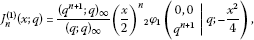(6)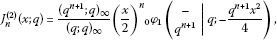(7)(8)

where $φ s r$ is the basic hypergeometric function 

$φ s r ( a 1 , … , a r b 1 , … , b s | q ; z ) = ∑ k = 0 ∞ ( a 1 , … , a r ; q ) k ( b 1 , … , b s ; q ) k ( ( − 1 ) k q k 2 ( k − 1 ) ) 1 + s − r z k ( q ; q ) k .$
(9)

The functions $J n ( i ) (x;q)$, $i=1,2$, are q-analogues of the Bessel function, and the function $J n ( 3 ) (x;q)$ is a q-analogue of the modified Bessel function.

Rogov [7, 8] introduced generalized modified q-Bessel functions, similarly to the classical case , as

$I n i (x;q)= ( q n + 1 ; q ) ∞ ( q ; q ) ∞ ( x 2 ) n δ φ 1 ( 0 , 0 , … , 0 q n + 1 | q ; q ( n + 1 ) ( 2 − δ ) 2 x 2 4 ) ,$

where

Recently, Mansour and et al.  studied the following q-Bessel function:

$J n ( 4 ) (x;q)= ( x / 2 ) n ( q ; q ) n 0 φ 2 ( − 0 , q n + 1 | q ; − q 3 ( n + 1 ) 2 x 2 4 ) ,$

which is a q-analogy of the modified Bessel function.

In this paper, we define the generalized q-Bessel function and study some of its properties. Also, in analogy with the ordinary Lie theory [11, 12], we derive algebraically the generating function of the generalized q-Bessel function.

## 2 The generalized q-Bessel function and its generating function

Definition 2.1 The generalized q-Bessel function is defined by

$J n (x,a;q)= ( x / 2 ) n ( q ; q ) n ∑ k = 0 ∞ ( − 1 ) k ( a + 1 ) q a k 2 ( k + n ) ( q n + 1 ; q ) k ( x 2 / 4 ) k ( q ; q ) k ,$
(10)

which converges absolutely for all x when $a∈ Z +$ and for $|x|<2$ if $a=0$.

As special cases of $J n (x,a;q)$, we get

$J n ( 1 ) ( x ; q ) = J n ( x , 0 ; q ) , J n ( 2 ) ( x ; q ) = J n ( x , 2 ; q ) , J n ( 3 ) ( x ; q ) = J n ( x , 1 ; q ) , J n ( 4 ) ( x ; q ) = J n ( x , 3 ; q ) .$

Lemma 2.2 The function $J n (x,a;q)$ is a q-analogy of each of the Bessel function and the modified Bessel function.

Proof

$lim q → 1 J n ( ( 1 − q ) x , a ; q ) = lim q → 1 { ( 1 − q ) n ( q , q ) n ( x 2 ) n ∑ k = 0 ∞ ( − 1 ) k ( a + 1 ) q a k ( k + n ) 2 ( 1 − q ) 2 k ( q n + 1 ; q ) k ( q ; q ) k ( x 2 ) 2 k } = 1 ( 1 ) n ( x 2 ) n ∑ k = 0 ∞ ( − 1 ) k ( a + 1 ) ( n + 1 ) k ( 1 ) k ( x 2 ) 2 k = ( x 2 ) n ∑ k = 0 ∞ ( − 1 ) k ( a + 1 ) Γ ( n + k + 1 ) Γ ( k + 1 ) ( x 2 ) 2 k .$

Hence, we get

$lim q → 1 J n ( ( 1 − q ) x , a ; q ) = J n (x);a=0,2,4,…$
(11)

and

$lim q → 1 J n ( ( 1 − q ) x , a ; q ) = I n (x);a=1,3,5,…,$
(12)

where $J n (x)$ is the Bessel function and $I n (x)$ is the modified Bessel function. □

Lemma 2.3 The function $J n (x,a;q)$ satisfies

$J − n (x,a;q)= ( − 1 ) n ( a + 1 ) J n (x,a;q),n∈Z.$
(13)

Proof Using the definition (10), we get

$J − n (x,a;q)= ∑ k = n ∞ ( − 1 ) k ( a + 1 ) q a k ( k − n ) 2 ( q − n + k + 1 ; q ) ∞ ( q ; q ) ∞ ( q ; q ) k ( x 2 ) 2 k − n .$

For $s=k−n$, we obtain

$J − n (x,a;q)= ∑ s = 0 ∞ ( − 1 ) ( s + n ) ( a + 1 ) q a s ( s + n ) 2 ( q s + 1 ; q ) ∞ ( q ; q ) ∞ ( q ; q ) s + n ( x 2 ) 2 s + n ,$

and using the relations 

$( q s + 1 ; q ) ∞ = ( q n + s + 1 ; q ) ∞ ( q s + 1 ; q ) n , ( q ; q ) s + n = ( q ; q ) s ( q s + 1 ; q ) n ,$

we obtain

$J − n ( x , a ; q ) = ( − 1 ) n ( a + 1 ) ∑ s = 0 ∞ ( − 1 ) s ( a + 1 ) q a s ( s + n ) 2 ( q n + s + 1 ; q ) ∞ ( q ; q ) ∞ ( q ; q ) s ( x 2 ) 2 s + n = ( − 1 ) n ( a + 1 ) J n ( x , a ; q ) .$

□

Lemma 2.4 The function $J n (x,a;q)$ satisfies the relation

$J n (−x,a;q)= ( − 1 ) n J n (x,a;q),n∈Z,$
(14)

and hence it is even (or odd) function if the integer n is even (or odd).

Now we will deduce the generating function of the generalized q-Bessel function $J n (x,a;q)$.

Theorem 1 The generating function $g(x,t,a;q)$ of the function $J n (x,a;q)$ is given by

$g(x,t,a;q)= E q ( q a 4 x t 2 , a 2 ) E q ( ( − 1 ) a + 1 q a 4 x 2 t , a 2 ) = ∑ n = − ∞ ∞ q a n 2 4 J n (x,a;q) t n .$
(15)

Proof

Let

$g(x,t,a;q)= E q ( q a 4 x t 2 , a 2 ) E q ( ( − 1 ) a + 1 q a 4 x 2 t , a 2 ) ,$

then

$g(x,t,a;q)= ∑ r = 0 ∞ ∑ s = 0 ∞ ( − 1 ) s ( a + 1 ) q a 2 [ ( r 2 ) + ( s 2 ) ] + a 4 ( s + r ) ( q ; q ) r ( q ; q ) s ( x 2 ) s + r t r − s .$

For $s=r−n$, we get

$g(x,t,a;q)= ∑ n = − ∞ ∞ ∑ r = 0 ∞ ( − 1 ) ( r − n ) ( a + 1 ) q a 2 [ ( r 2 ) + ( r − n 2 ) ] + a 4 ( 2 r − n ) ( q ; q ) r ( q ; q ) r − n ( x 2 ) 2 r − n t n .$

Hence, for $n≤0$, the coefficient of $t n$ is given by

$c n = ( − 1 ) − n ( a + 1 ) q a n 2 4 ( x / 2 ) − n ( q ; q ) − n ∑ r = 0 ∞ ( − 1 ) r ( a + 1 ) q a r 2 ( r − n ) ( q ; q ) r ( q − n + 1 ; q ) r ( x 2 ) 2 r ,$

where $( q , q ) r − n = ( q ; q ) − n ( q − n + 1 ; q ) r$ for $n≤0$. Then

$c n = ( − 1 ) − n ( a + 1 ) q a n 2 4 J − n (x,a;q)= q a n 2 4 J n (x,a;q).$

Similarly, for $n≥0$. □

As special cases of $g n (x,t,a;q)$, we obtain

$g n (x,t,0;q)= E q ( x t 2 , 0 ) E q ( − x 2 t , 0 ) = e q ( x t 2 ) e q ( − x 2 t ) ,$
(16)

which is a generating function of the q-Bessel function $J n ( 1 ) (x;q)$ ,

$g n ( x , t q , 2 ; q ) = E q ( x t 2 , 1 ) E q ( − q x 2 t , 1 ) = E q ( x t 2 ) E q ( − q x 2 t ) ,$
(17)

which is a generating function of the q-Bessel function $J n ( 2 ) (x;q)$ ,

$g n (x,t,1;q)= E q ( q 4 x t 2 , 1 2 ) E q ( q 4 x 2 t , 1 2 ) ,$
(18)

which is a generating function of the q-Bessel function $J n ( 3 ) (x;q)$ and

$g n (x,t,3;q)= E q ( q 3 / 4 x t 2 , 3 2 ) E q ( q 3 / 4 x 2 t , 3 2 ) ,$
(19)

which is a generating function of the q-Bessel function $J n ( 4 ) (x;q)$ .

## 3 The q-difference equation of the function $J n (x,a;q)$

Now the generating function method  will be used to deduce the q-difference equation of the generalized q-Bessel function. Using equation (15), we have

$E q ( q a 4 x t h 2 , a 2 ) E q ( ( − 1 ) a + 1 q a 4 x h 2 t , a 2 ) = ∑ n = − ∞ ∞ q a n 2 4 J n (xh,a;q) t n ;h∈R−{0}.$
(20)

By applying the operator $D q$, we get

$( − 1 ) a + 1 q a 4 h 2 ( 1 − q ) t E q ( q a + 4 4 x t h 2 , a 2 ) E q ( ( − 1 ) a + 1 q 3 a 4 x h 2 t , a 2 ) + q a 4 t h 2 ( 1 − q ) E q ( q 3 a 4 x t h 2 , a 2 ) E q ( ( − 1 ) a + 1 q a 4 x h 2 t , a 2 ) = ∑ n = − ∞ ∞ q a n 2 4 D q J n ( x h , a ; q ) t n .$

Using equation (20), we obtain

$( − 1 ) a + 1 q a 4 + a 2 ( n + 1 2 ) + n + 1 2 2 ( 1 − q ) J n + 1 ( q a + 2 4 x h , a ; q ) + q a 4 + a n ( n − 1 ) 4 2 ( 1 − q ) J n − 1 ( q a 4 x h , a ; q ) = q a n 2 4 h D q J n ( x h , a ; q ) .$

Hence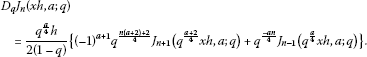(21)

Similarly, we can prove the following relation: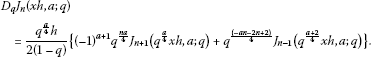(22)

By using equations (21) and (22), we obtain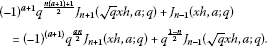(23)

But

$J n − 1 ( q x h , a ; q ) = ( q x h 2 ) n − 1 ( q ; q ) ∞ ∑ k = 0 ∞ ( − 1 ) k ( a + 1 ) q a k 2 ( k + n − 1 ) ( q n + k ; q ) ∞ ( q ; q ) k ( q x h 2 ) 2 k = q n − 1 2 ( x h 2 ) n − 1 ( q ; q ) ∞ ∑ k = 0 ∞ ( − 1 ) k ( a + 1 ) q a k 2 ( k + n − 1 ) ( q n + k ; q ) ∞ ( q ; q ) k ( q k − 1 + 1 ) ( x h 2 ) 2 k = q n − 1 2 J n − 1 ( x h , a ; q ) − q n − 1 2 ( x h 2 ) n − 1 ( q ; q ) ∞ ∑ k = 0 ∞ ( − 1 ) ( k + 1 ) ( a + 1 ) q a ( k + 1 ) ( k + n ) 2 ( q ; q ) k × ( q n + k + 1 ; q ) ∞ ( x h 2 ) 2 k + 2 = q n − 1 2 { J n − 1 ( x h , a ; q ) + ( − 1 ) a q a n 2 x h 2 J n ( q a 4 x h , a ; q ) } .$

Then

$q 1 − n 2 J n − 1 ( q xh,a;q)− J n − 1 (xh,a;q)= ( − 1 ) a q a n 2 x h 2 J n ( q a 4 x h , a ; q ) .$
(24)

Equations (23) and (24) give the relation

$q 1 + n 2 J n + 1 ( q xh,a;q)− J n + 1 (xh,a;q)= − x h 2 J n ( q a 4 x h , a ; q ) .$
(25)

Equations (21) and (25) give the relation

${ D q + q a ( 1 − n ) 4 ( 1 − q ) x [ q n 2 δ q − 1 ] } J n (xh,a;q)= ( − 1 ) a + 1 h q ( a + 2 ) ( n + 1 ) 4 2 ( 1 − q ) J n + 1 ( q a + 2 4 x h , a ; q ) ,$
(26)

where the operator $δ q$ is given by $δ q f(x)=f( q x)$.

Now consider the following operator:

$M n , q = { D q + q a ( 1 − n ) 4 ( 1 − q ) x [ q n 2 δ q − 1 ] } ,$
(27)

then we can rewrite equation (26) by the formula

$M n , q J n (xh,a;q)= ( − 1 ) a + 1 h q ( a + 2 ) ( n + 1 ) 4 2 ( 1 − q ) J n + 1 ( q a + 2 4 x h , a ; q ) .$
(28)

Also, equations (22) and (24) give the relation

${ D q + q − a ( 1 + n ) 4 ( 1 − q ) x [ q − n 2 δ q − 1 ] } J n (xh,a;q)= h q ( a + 2 ) ( 1 − n ) 4 2 ( 1 − q ) J n − 1 ( q a + 2 4 x h , a ; q ) .$
(29)

If we consider the operator

$N n , q = { D q + q − a ( 1 + n ) 4 ( 1 − q ) x [ q − n 2 δ q − 1 ] } ,$
(30)

then we can rewrite equation (29) by the formula

$N n , q J n (xh,a;q)= h q ( a + 2 ) ( 1 − n ) 4 2 ( 1 − q ) J n − 1 ( q a + 2 4 x h , a ; q ) .$
(31)

Hence, the q-difference equation of the function $J n (x,a;q)$ takes the formula

$M n − 1 , q N n , q J n (xh,a;q)= ( − 1 ) a + 1 q a + 2 4 h 2 4 ( 1 − q ) 2 J n ( q a + 2 2 x h , a ; q ) .$
(32)

If we replace h by $1−q$ and consider the limit as q tends to 1, then we obtain

$( 1 2 d d x − n − 1 2 x ) ( 1 2 d d x + n 2 x ) y(x)= ( − 1 ) a + 1 4 y(x),$
(33)

or

$x 2 y ″ (x)+x y ′ − ( n 2 + ( − 1 ) a + 1 x 2 ) y=0.$
(34)

The differential equation (34) gives the Bessel function at $a=0,2,4,…$ and the modified Bessel function at $a=1,3,5,…$ , which proves again that $J n (x,a;q)$ is a q-analogy of each of them.

## 4 The recurrence relations of the function $J n (x,a;q)$

Lemma 4.1

$J n (x,a;q)= 2 x ( 1 − q n + 1 ) J n + 1 ( q − a / 4 x , a ; q ) + ( − 1 ) a + 1 q ( a + 2 ) ( n + 1 ) 2 J n + 2 (x,a;q).$
(35)

Proof

$J n ( x , a ; q ) = ( x / 2 ) n ( q ; q ) n ∑ k = 0 ∞ ( − 1 ) k ( a + 1 ) q a k 2 ( k + n ) ( q ; q ) k ( q n + 1 ; q ) k + 1 ( 1 − q n + 1 + q n + 1 − q n + k − 1 ) ( x 2 4 ) k = 2 x ( 1 − q n + 1 ) ( x / 2 ) n + 1 ( q ; q ) n + 1 ∑ k = 0 ∞ ( − 1 ) k ( a + 1 ) q a k 2 ( k + n + 1 ) ( q ; q ) k ( q n + 2 ; q ) k ( ( q − a 4 x ) 2 4 ) k + q n + 1 ( x / 2 ) n + 2 ( q ; q ) n + 2 ∑ k = 1 ∞ ( − 1 ) k ( a + 1 ) q a k 2 ( k + n ) ( q ; q ) k − 1 ( q n + 3 ; q ) k − 1 ( x 2 4 ) k − 1 = 2 x ( 1 − q n + 1 ) J n + 1 ( q − a / 4 x , a ; q ) + ( − 1 ) a + 1 q ( a + 2 ) ( n + 1 ) 2 ( x / 2 ) n + 2 ( q ; q ) n + 2 ∑ k = 0 ∞ ( − 1 ) k ( a + 1 ) q a k 2 ( k + n + 2 ) ( q ; q ) k ( q n + 3 ; q ) k ( x 2 4 ) k = 2 x ( 1 − q n + 1 ) J n + 1 ( q − a / 4 x , a ; q ) + ( − 1 ) a + 1 q ( a + 2 ) ( n + 1 ) 2 J n + 2 ( x , a ; q ) .$

□

Similarly, if we write $(1− q k + q k − q n + k − 1 )$ instead of $(1− q n + 1 + q n + 1 − q n + k − 1 )$, we can prove the following lemma.

Lemma 4.2

$J n (x,a;q)= 2 x ( 1 − q n + 1 ) J n + 1 ( q 2 − a 4 x , a ; q ) + ( − 1 ) a + 1 q a ( n + 1 ) 2 J n + 2 (x,a;q).$
(36)

Now, if we replace a by $a+2$ in the recurrence relation (36), we get the recurrence relation (35). Then we have the following lemma.

Lemma 4.3 The two functions $J n (x,a;q)$ and $J n (x,a+2;q)$ have the same recurrence relation.

Then we have two cases of the recurrence relation.

Case (1): The function $J n (x,a;q)$ has the recurrence relation

$J n (x,a;q)= 2 x ( 1 − q n + 1 ) J n + 1 (x,a;q)− q n + 1 J n + 2 (x,a;q);∀a=0,2,4,…,$
(37)

which is the recurrence relation of each of $J n ( 1 ) (x;q)$ and $J n ( 2 ) (x;q)$.

Case (2): The function $J n (x,a;q)$ has the recurrence relation

$J n ( x , a ; q ) = q n 4 [ 2 x ( 1 − q n + 1 ) − q n 2 x 2 ] J n + 1 ( x , a ; q ) + q n + 1 / 2 J n + 2 ( x , a ; q ) ; ∀ a = 1 , 3 , 5 , … ,$
(38)

which is the recurrence relation of each of $J n ( 3 ) (x;q)$ and $J n ( 4 ) (x;q)$.

## 5 The quantum algebra approach to $J n (x,a;q)$

The quantum algebra $E q (2)$ is determined by generators H, $E +$ and $E −$ with the commutation relations

$[H, E + ]= E + ,[H, E − ]=− E − ,[ E − , E + ]=0.$
(39)

By considering the irreducible representations $(ω)$ of $E q (2)$ characterized by $ω∈C$, then the spectrum of the operator H will be the set of integers , and the basis vectors $f m$, $m∈Z$, satisfy

$E ± f m =ω f m ± 1 ,H f m =m f m , E + E − f m = ω 2 f m ,$
(40)

where $C= E + E −$ is the Casimir operator which commutes with the generators H, $E +$ and $E −$. The following differential operators presented a simple realization of $(ω)$

$H=z d d z , E + =ωz, E − = ω z$
(41)

acting on the space of all linear combinations of the functions $z m$, z a complex variable, $m∈Z$, with basis vectors $f m (z)= z m$.

In the ordinary Lie theory, matrix elements $T s m$ of the complex motion group in the representation $(ω)$ are typically defined by the expansions 

$e α E + e β E − e τ H f m = ∑ s = − ∞ ∞ T s m (α,β,τ) f s .$
(42)

If we replace the mapping $e x$ by the mapping $E q (x,a/2)$ from the Lie algebra to the Lie group with putting $τ=0$ in equation (42), we can use the model (41) to find the following q-analog of matrix elements of $(ω)$:

$E q (α E + ,a/2) E q (β E − ,a/2) f m = ∑ s = − ∞ ∞ T s m (α,β) f s ,$
(43)

and hence

$E q ( α ω z , a 2 ) E q ( β ω z , a 2 ) z m = ∑ r , t = 0 ∞ q a 2 [ ( r 2 ) + ( t 2 ) ] ( q ; q ) r ( q ; q ) t ω r + t α r β t z m − t + r .$

Now replace s by $m−t+r$ to get

$E q ( α ω z , a 2 ) E q ( β ω z , a 2 ) z m = ∑ s = − ∞ ∞ ∑ r = 0 ∞ q a 2 [ ( r 2 ) + ( m + r − s 2 ) ] ( q ; q ) r ( q ; q ) m + r − s ω m + 2 r − s α r β m + r − s z s$

and by equating the coefficient of $z s$ for $m≥s$ on both sides, we get

$T s m ( α , β ) = q a 2 ( m − s 2 ) ( q ; q ) m − s ( ω β ) m − s ∑ r = 0 ∞ q a 2 r ( r + m − s − 1 ) ( q ; q ) r ( q m − s + 1 ; q ) r ( ω 2 α β ) r , m ≥ s = q a 4 ( m − s ) 2 ( ( − 1 ) − ( a + 1 ) β α ) m − s 2 ( − 1 ) ( m − s ) ( a + 1 ) J m − s ( 2 q − a 4 ω ( − 1 ) a + 1 α β , a ; q ) , ( − 1 ) a + 1 α β > 0 ; m ≥ s = q a 4 ( s − m ) 2 ( ( − 1 ) ( a + 1 ) α β ) s − m 2 J s − m ( 2 q − a 4 ω ( − 1 ) a + 1 α β , a ; q ) , ( − 1 ) a + 1 α β > 0 ; m ≥ s ,$

where $J − n (x,a;q)= ( − 1 ) n ( a + 1 ) J n (x,a;q)$.

Similarly,

$T s m ( α , β ) = q a 4 ( s − m ) 2 ( ( − 1 ) ( a + 1 ) α β ) s − m 2 J s − m ( 2 q − a 4 ω ( − 1 ) a + 1 α β , a ; q ) , ( − 1 ) a + 1 α β > 0 ; s ≥ m .$

The combination between the two cases gives us the following expression:

$T s m ( α , β ) = q a 4 ( s − m ) 2 ( ( − 1 ) ( a + 1 ) α β ) s − m 2 J s − m ( 2 q − a 4 ω ( − 1 ) a + 1 α β , a ; q ) , ( − 1 ) a + 1 α β > 0 ,$
(44)

which is valid for all $m,s∈Z$. Then we get the following result.

Lemma 5.1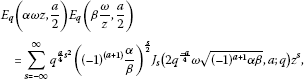(45)

where $( − 1 ) a + 1 αβ>0$.

As special cases:

Considering (45) with $a=0$, $α=−β=1$, $z=t$ and $ω= x 2$, we obtain the relation (16).

Considering (45) with $a=2$, $α=−β=1$, $z= t q$ and $ω= q x 2$, we obtain the relation (17).

Considering (45) with $a=1$, $α=β=1$, $z=t$ and $ω= q 4 x 2$, we obtain the relation (18).

Considering (45) with $a=3$, $α=β=1$, $z=t$ and $ω= q 3 / 4 x 2$, we obtain the relation (19).

## References

1. 1.

Gasper G, Rahman M: Basic Hypergeometric Series. 2nd edition. Cambridge University Press, Cambridge; 2004.

2. 2.

Floreanini R, LeTourneux J, Vinet L: More on the q -oscillator algebra and q -orthogonal polynomials. J. Phys. A, Math. Gen. 1995, 28: L287-L293. 10.1088/0305-4470/28/10/002

3. 3.

Exton H: q-Hypergeometric Functions and Applications. Ellis Horwood, Chichester; 1983.

4. 4.

Atakishiyev NM: On a one-parameter family of q -exponential functions. J. Phys. A, Math. Gen. 1996, 29: L223-L227. 10.1088/0305-4470/29/10/001

5. 5.

Jackson FH: On q -functions and certain difference operator. Trans. R. Soc. Edinb. 1908, 46: 253–281.

6. 6.

Jackson FH: The application of basic numbers to Bessel’s and Legendre’s functions. Proc. Lond. Math. Soc. 1903–1904, 2: 192–220.

7. 7.

Rogov, V-BK: q-Bessel Macdonald functions. arXiv:math.QA/0010170v1, 17 Oct. 2000

8. 8.

Rogov, V-BK: The integral representations of the q-Bessel Macdonald functions. arXiv:math.QA/0101259v1, 31 Jan. 2001

9. 9.

Erdélyi A, Magnus W, Oberhettinger F, Tricomi FG 2. In Higher Transcendental Functions. McGraw-Hill, New York; 1953. (based, in part, on notes left by Harry Bateman)

10. 10.

Mansour M, Al-Shomarani MM: New q -analogy of modified Bessel function and the quantum algebra $E q (2)$ . J. Comput. Anal. Appl. 2013, 15(4):655–664.

11. 11.

Miller W: Lie Theory and Special Functions. Academic Press, San Diego; 1968.

12. 12.

Kalnins EG, Mukherjee S, Miller W: Models of q -algebra representations: the group of plane motions. SIAM J. Math. Anal. 1994, 25: 513–527. 10.1137/S0036141092224613

13. 13.

Dattoli G, Torre A: q -Bessel functions: the point of view of the generating function method. Rend. Mat. Appl. (7) 1997, 17: 329–345.

## Author information

Authors

### Corresponding author

Correspondence to Mansour Mahmoud.

### Competing interests

The author declares that he has no competing interests.

### Author’s contributions

The author read and approved the final manuscript.

## Rights and permissions

Reprints and Permissions

Mahmoud, M. Generalized q-Bessel function and its properties. Adv Differ Equ 2013, 121 (2013). https://doi.org/10.1186/1687-1847-2013-121